# 嘉陵江梯级水库运行期设计洪水及汛控水位Design Floods and Control Water Levels of Jialing River Cascade Reservoirs in Operation Period

DOI: 10.12677/JWRR.2019.83025, PDF, HTML, XML, 下载: 372  浏览: 551  国家自然科学基金支持

Abstract: The differences between reservoir construction and operation periods, flood limited water level and flood control water level, were discussed. The most likely regional flood composition method was applied to derive and analyze design floods as well as the flood control water levels of Jialing River cascade reservoirs in operation period. Results reveal that: 1) the design floods of downstream reservoirs are moderately influenced by the operation of upstream reservoirs. The 1000-year design flood peak, 3 d, and 7 d flood volumes of Caojie Reservoir decrease by 11,456 m3/s (18%), 1.35 (12%) and 1.11 billion m3 (5%), respectively; 2) the flood control water levels of downstream reservoirs are slightly higher than flood limited water level with the same flood prevention standards. The flood control (limited) water levels of Tingzikou and Caojie Reservoirs in operation period are 447.4 (447) and 202.3 (200) m, which can increase the hydropower generation by 1.1% and 4.8%, respectively, and in total 0.11 billion KW∙h annually.

1. 引言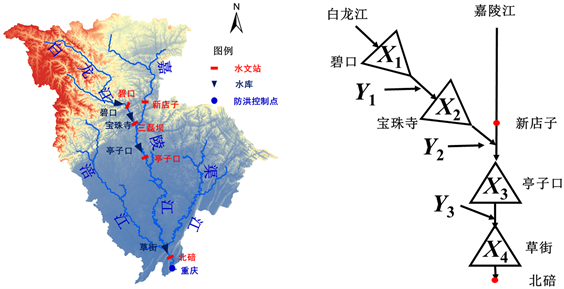Figure 1. Sketch map of cascade reservoirs and the Jialing River basin

2. 最可能地区组成法

$\begin{array}{l}\mathrm{max}\text{\hspace{0.17em}}\text{\hspace{0.17em}}\text{\hspace{0.17em}}f\left({x}_{1},{x}_{2},{x}_{3},{x}_{4}\right)=c\left({u}_{1},{u}_{2},{u}_{3},{u}_{4}\right)\underset{i=1}{\overset{4}{\prod }}{f}_{{X}_{i}}\left({x}_{i}\right)\\ \begin{array}{cc}\text{s}\text{.t}\text{.}& \begin{array}{l}\text{\hspace{0.17em}}\text{\hspace{0.17em}}{x}_{1}+{y}_{1}={x}_{2}\\ \text{\hspace{0.17em}}\text{\hspace{0.17em}}{x}_{1}+{y}_{1}+{y}_{2}={x}_{3}\\ \text{\hspace{0.17em}}\text{\hspace{0.17em}}{x}_{1}+{y}_{1}+{y}_{2}+{y}_{3}={x}_{4}\end{array}\end{array}\end{array}\right\}$ (1)

$C\left(u;\Sigma ,v\right)={\int }_{-\infty }^{{\Phi }^{-1}\left({u}_{1}\right)}\cdots {\int }_{-\infty }^{{\Phi }^{-1}\left({u}_{4}\right)}\frac{\Gamma \left(\frac{v+4}{v}\right)}{\Gamma \left(\frac{v}{2}\sqrt{{\left(\pi v\right)}^{4}|\Sigma |}\right)}{\left(1+\frac{1}{v}{\omega }^{\text{T}}{\Sigma }^{-1}\omega \right)}^{\frac{v+4}{2}}\text{d}\omega$ (2)

1) 根据样本确定各分区的边缘分布和联合分布。

2) 考虑水量平衡约束，以最小化联合概率密度函数的负值为目标函数进行优化求解：

$\begin{array}{l}\begin{array}{cc}\mathrm{min}& -\end{array}f\left({x}_{1},{x}_{2},{x}_{3},{x}_{4}\right)=-c\left({u}_{1},{u}_{2},{u}_{3},{u}_{4}\right)\underset{i=1}{\overset{4}{\prod }}{f}_{{X}_{i}}\left({x}_{i}\right)\\ \begin{array}{cc}\text{s}\text{.t}\text{.}& \begin{array}{l}\text{\hspace{0.17em}}\text{\hspace{0.17em}}{x}_{1}+{y}_{1}={x}_{2}\\ \text{\hspace{0.17em}}\text{\hspace{0.17em}}{x}_{1}+{y}_{1}+{y}_{2}={x}_{3}\\ \text{\hspace{0.17em}}\text{\hspace{0.17em}}{x}_{1}+{y}_{1}+{y}_{2}+{y}_{3}={x}_{4}=z\end{array}\end{array}\end{array}\right\}$ (3)

3) 由求解得到的最优频率组合及各分区的边缘分布推求最可能地区组成。

3. 实例研究Table 1. List of design flood characteristic values of cascade reservoirs in the Jialing River

3.1. 各分区洪水的边缘分布Table 2. Estimated parameters of marginal distributions for each sub-basin

3.2. 各分区洪水的联合分布Table 3. Goodness-of-fit of estimated t-Copula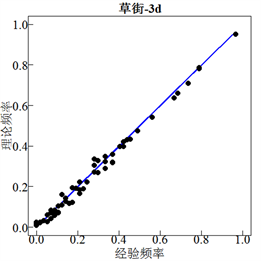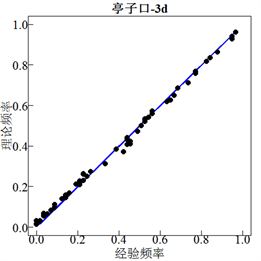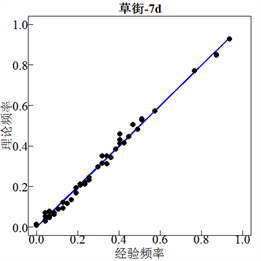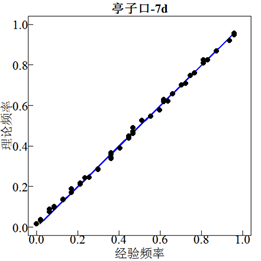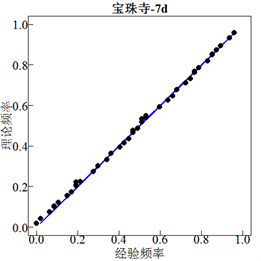Figure 2. The P-P plots fitted by the joint distributions of 3 d and 7 d annual maximum flood volumes of composed sub-basins

3.3. 洪水地区组成和梯级水库调蓄影响Table 4. Results of most likely and equivalent frequency composition at Caojie Reservoir/(108 m3)Table 5. Comparison of design peak discharges regulated by upper cascade reservoirs at Caojie Reservoir/(m3∙s−1)

3.4. 梯级水库运行期设计洪水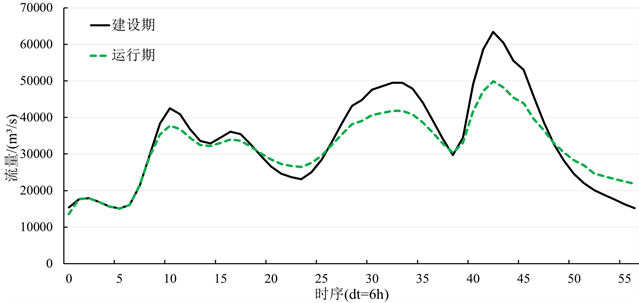Figure 3. Comparison of 1000-year design flood hydrographs between construction and operation periods of Caojie Reservoir

4. 结论

1) 最可能地区组成结果合理可信，能够为洪水地区组成的分析计算提供参考；

2) 梯级水库联合调度对下游洪水具有一定的削减作用，草街水库运行期千年一遇的设计洪峰、3 d和7 d洪量的削减量(削减率)分别为11,457 m3/s (18%)、13.51亿m3 (12%)和11.12亿m3 (5%)；

3) 在保证防洪标准不变的前提下，亭子口与草街梯级水库运行期汛控水位相比汛限水位可适当抬高，分别为447 m (447.4 m)和200 m (202.3 m)，年均发电量分别增加了1.1%和4.8%，共增发电量1.1亿kW∙h/年；

4) 若预测到流域超标准洪水或发生一些特殊紧急情况，则按建设期汛限水位指导水库调度运行。Table 6. Comparison of 1000-year design flood values between construction and operation periods

  水利部. 中国水利统计年鉴2017 [M]. 北京: 中国水利水电出版社, 2017: 29-34. Ministry of Water Resources. China water statistical yearbook 2017. Beijing: Hydropower and Electrical Press, 2017: 29-34 (in Chinese)  水利部. 水利水电工程设计洪水计算规范(SL44-2006) [S]. 北京: 中国水利水电出版社, 2006. Ministry of Water Resources. Design flood calculation regulating for water resources and hydropower engineering. Beijing: Hydropower and Electrical Press, 2006. (in Chinese)  郭生练, 熊丰, 尹家波, 陈柯兵. 水库运用期设计洪水理论和方法[J]. 水资源研究, 2018, 7(4): 327-339. GUO Shenglian, XIONG Feng, YIN Jiabo and CHEN Kebing. Theory and method of design flood in reservoir operation period. Journal of Water Resources Research, 2018, 7(4): 327-339. (in Chinese)  闫宝伟, 郭生练, 郭靖, 陈璐, 刘攀, 陈华. 基于Copula函数的设计洪水地区组成研究[J]. 水力发电学报, 2010, 29(6): 60-65. YAN Baowei, GUO Shenglian, GUO Jing, CHEN Lu, LIU Pan and CHEN Hua. Regional design flood composition based on Copula function. Journal of Hydroelectric Engineering, 2010, 29(6): 60-65. (in Chinese)  刘章君, 郭生练, 李天元, 徐长江. 梯级水库设计洪水最可能地区组成法计算通式[J]. 水科学进展, 2014, 25(4): 575-584. LIU Zhangjun, GUO Shenglian, LI Tianyuan and XU Changjiang. General formula derivation of most likely regional composition method for design flood estimation of cascade reservoirs system. Advances in Water Science, 2014, 25(4): 575-584. (in Chinese)  熊丰, 郭生练, 陈柯兵, 等. 金沙江下游梯级水库运用期设计洪水[J]. 水科学进展, 2019. http://kns.cnki.net/kcms/detail/32.1309.p.20190417.1529.024.html XIONG Feng, GUO Shenglian, CHEN Kebing, et al. Design flood for cascade reservoirs during operation period in the downstream Jinsha River. Advances in Water Science, 2019. http://kns.cnki.net/kcms/detail/32.1309.p.20190417.1529.024.html (in Chinese)  程中阳, 张行南, 夏达忠. 基于ECMWF和WRF的嘉陵江流域面雨量预报精度评估[J]. 长江科学院院报, 2018, 35(11): 24-29. CHENG Zhongyang, ZHANG Xingnan and XIA Dazhong. Evaluation of areal rainfall forecast precision in Jialing River basin by using ECMWF and WRF Meteorological Products. Journal of Yangtze River Scientific Research Institute, 2018, 35(11): 24-29. (in Chinese)  程中阳, 张行南, 方园皓. 集水面积阈值确定方法在嘉陵江流域的比较应用[J]. 人民长江, 2017, 48(15): 25-29. CHENG Zhongyang, ZHANG Xingnan and FANG Yuanhao. Application and comparison of identification methods for critical catchment area threshold in Jialing River Basin. Yangtze River, 2017, 48(15): 25-29. (in Chinese)